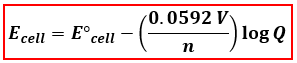# Problem: A voltaic cell consists of a Pb/ Pb2+ half-cell and a Cu/Cu2+ half-cell at 25°C. The initial concentrations of Pb2+ and Cu2+ are 0.0500 M and 1.50 M, respectively.a. What is the initial cell potential?

###### FREE Expert Solution
87% (212 ratings)
###### FREE Expert Solution

We are asked to find the initial cell potential in the voltaic cell given. We will use the Nernst Equation to calculate the cell potential. The Nernst Equation relates the concentrations of compounds and cell potential.Ecell = cell potential under non-standard conditions
cell = standard cell potential
n = number of e- transferred
Q= reaction quotient = [products]/[reactants]

In the Nernst Equation, the E°cell is needed but only Ecell was given. We have to determine the E°cell first and as well as the anode (oxidation) and cathode (reduction) in the concentration cell and the number of electrons transferred (n).

87% (212 ratings)###### Problem Details

A voltaic cell consists of a Pb/ Pb2+ half-cell and a Cu/Cu2+ half-cell at 25°C. The initial concentrations of Pb2+ and Cu2+ are 0.0500 M and 1.50 M, respectively.

a. What is the initial cell potential?

Frequently Asked Questions

What scientific concept do you need to know in order to solve this problem?

Our tutors have indicated that to solve this problem you will need to apply the The Nernst Equation concept. You can view video lessons to learn The Nernst Equation. Or if you need more The Nernst Equation practice, you can also practice The Nernst Equation practice problems.

What is the difficulty of this problem?

Our tutors rated the difficulty ofA voltaic cell consists of a Pb/ Pb2+ half-cell and a Cu/Cu2...as high difficulty.

How long does this problem take to solve?

Our expert Chemistry tutor, Jules took 4 minutes and 35 seconds to solve this problem. You can follow their steps in the video explanation above.

What professor is this problem relevant for?

Based on our data, we think this problem is relevant for Professor Marville's class at UIUC.

What textbook is this problem found in?

Our data indicates that this problem or a close variation was asked in Chemistry: A Molecular Approach - Tro 3rd Edition. You can also practice Chemistry: A Molecular Approach - Tro 3rd Edition practice problems.